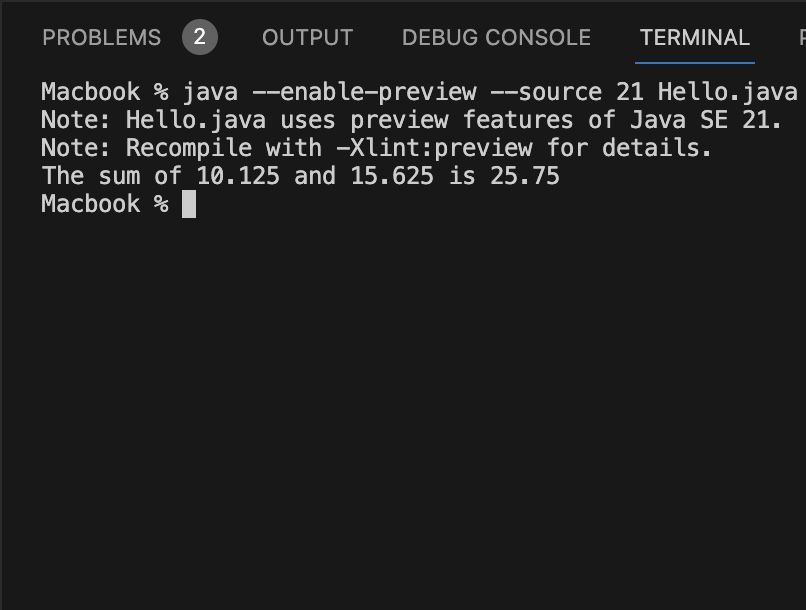# [JEP 430] Java JDK 21 New Feature - String Templates (Preview)

Hello Java Developer!

Welcome to the Java JDK 21 Series where we cover all the new features that are introduced in this latest LTS Version.

## Setting up the Stage:

Let's say you add two numbers in Python and you want to print the output on the console using print() function. To achieve this we can make use of something called as String Interpolation.

``````num1 = 10.125
num2 = 11.525

sum = num1 + num2

print(f"The sum of {num1:} and {num2} is {sum:.3f}")``````

Result:

The sum of 10.125 and 11.525 is 21.65

Now if you want to do something similar in other languages that support string interpolation we be like below.

LanguageEquivalent String Interpolation
C#`\$"The sum of {num1} and {num2} is {num1 + num2:F2}"`
Visual Basic`\$"The sum of {num1} and {num2} is {num1 + num2:F2}"`
Python`f"The sum of {num1} and {num2} is {result:.2f}"`
Scala`s"The sum of \$num1 and \$num2 is \${num1 + num2}"`
Groovy`"The sum of \$num1 and \$num2 is \${num1 + num2}"`
Kotlin`"The sum of \$num1 and \$num2 is \${num1 + num2}"`
JavaScript`The sum of \${num1} and \${num2} is \${num1 + num2}`
Ruby`"The sum of #{num1} and #{num2} is #{num1 + num2}"`
Swift`"\(num1) plus \(num2) equals \(num1 + num2)"`
Go (Golang)`fmt.Sprintf("The sum of %v and %v is %.2f", num1, num2, num1+num2)`
Rust`format!("The sum of {} and {:.2} is {:.2}", num1, num2, num1 + num2);`

## How we deal with same Program in Java (prior to String Template)

1. Using String Concatenation Operator +
2. Example:

``````double result = num1 + num2;

NumberFormatter numberFormatter = new NumberFormatter();
String formattedResult = numberFormatter.format(result);

String result = "The sum of " + num1 + " and " + num2 + " is " + formattedResult;

class NumberFormatter {
public String format(double number) {
return String.format("%.2f", number);
}
}``````
3. Using MessageFormat
``````MessageFormat messageFormat = new MessageFormat("The sum of {0} and {1} equals {2}");
String resultString = messageFormat.format(num1, num2, num1 + num2);``````
4. Using StringBuilder
``````String stringResult = new StringBuilder()
.append("The Sum of ")
.append(num1)
.append(" and ")
.append(num2)
.append(" is ")
.append(num1 + num2)
.toString();``````
5. Using String::format
``String.format("The sum of %2\$d and %1\$d is %3\$d", num1, num2, num1 + num2);``
6. Using String::formatted
``"The sum of %2\$d and %1\$d is %3\$d".formatted(num1, num2, num1 + num2);``

## JEP 430 String Templates

As you saw in the above section, that most of the other programming languages supports string interpolation which is very convenient than concatenation. It makes the code more readable and provides more clarity especially when you have to deal with larger strings.

STR - Template expressions in JDK 21 are a new kind of expression in the Java programming language that can be used to perform string interpolation and can also turn structured text into any kind of object, according to domain-specific rules.

Example:

``````public class Hello {

public static void main(String... args) {
String name = "Sam";
System.out.println(STR."Hello, my name is \{name}");
}
}``````

```% java --enable-preview --source 21 Hello.java
Note: Hello.java uses preview features of Java SE 21.
Note: Recompile with -Xlint:preview for details.

Hello, my name is Sam```

Let's see the how with our sum of two numbers example works with Java String Template example.

``````public class Hello {

public static void main(String... args) {
double num1 = 10.125;
double num2 = 15.625;
System.out.println(STR."The sum of \{num1} and \{num2} is \{num1+num2}");
}
}``````

Output: The sum of 10.125 and 15.625 is 25.75

Note: You will not need to import anything to use the STR Template, it is a public static final field that is automatically imported into every Java source file.This is not an AI-generated article but is demonstrated by a human on an M1 Mac running Java JDK 21 (Initial Release) and macOS Sonoma 14.0.

Please support independent contributors like Code2care by donating a coffee.-

### Facing issues? Have Questions? Post them here! I am happy to answer!Author Info:

Rakesh is a seasoned developer with over 10 years of experience in web and app development, and a deep knowledge of operating systems. Author of insightful How-To articles for Code2care.